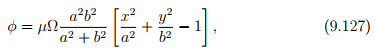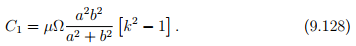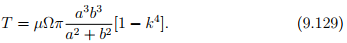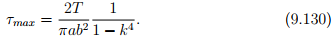# Hollow Elliptical Section Civil Engineering (CE) Notes | EduRev

## Civil Engineering (CE) : Hollow Elliptical Section Civil Engineering (CE) Notes | EduRev

The document Hollow Elliptical Section Civil Engineering (CE) Notes | EduRev is a part of the Civil Engineering (CE) Course Advanced Solid Mechanics - Notes, Videos, MCQs & PPTs.
All you need of Civil Engineering (CE) at this link: Civil Engineering (CE)

Hollow elliptical section

Here we study the torsion of a bar with a hollow elliptical section as shown in figure 9.23 where the inner boundary is a scaled ellipse similar to that of the outer boundary. Since, the inner ellipse is a scaled outer ellipse, the Prandtl stress function obtained for solid ellipse cross section in section 9.3.1 is a constant at the inner surface. Hence, the boundary condition (9.118) holds and therefore this stress functionis a solution to this boundary value problem of twisting of a hollow elliptical bar. Now, the constant C1, the value of φ at the inner boundary is obtained as,Using (9.127) and (9.128) in (9.126), twisting moment is obtained asSince, the stress function is same as in the case of a solid elliptical section, the stress distribution is also identical as before. Therefore, the maximum shear stress occurs at x = 0, y = ±b and is given by,Before concluding this section we observe that this solution scheme can be applied to other cross sections whose inner boundary coincides with a contour line of the stress function in the corresponding solid section problem.

Offer running on EduRev: Apply code STAYHOME200 to get INR 200 off on our premium plan EduRev Infinity!

## Advanced Solid Mechanics - Notes, Videos, MCQs & PPTs

42 videos|61 docs

,

,

,

,

,

,

,

,

,

,

,

,

,

,

,

,

,

,

,

,

,

;